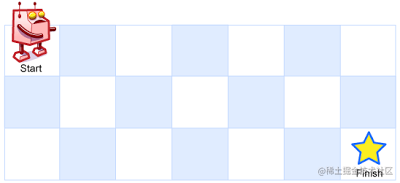LeetCode算法系列 62. 不同路径力扣原题

62. 不同路径1. 向右 -> 向下 -> 向下
2. 向下 -> 向下 -> 向右
3. 向下 -> 向右 -> 向下

方法一：动态规划

解题思路

$f(i,j)=f(i−1,j)+f(i,j−1)$

• 如果 $i=0$，那么 $f(0,j)$ =1；（为什么等于1，说白了只能走直线）
• 如果 $j=0$，那么 $f(i,0)$ =1；（为什么等于1，说白了只能走直线）
• 如果 $i=0$$j=0$，那么 $f(0,0)$ =1；（其实也能等于0，原地不动怎么定义而已）

代码实现

class Solution {
public int uniquePaths(int m, int n) {
int[][] f = new int[m][n];
for (int i = 0; i < m; ++i) {
f[i] = 1;
}
for (int j = 0; j < n; ++j) {
f[j] = 1;
}
for (int i = 1; i < m; ++i) {
for (int j = 1; j < n; ++j) {
f[i][j] = f[i - 1][j] + f[i][j - 1];
}
}
return f[m - 1][n - 1];
}
}

复杂度分析

• 时间复杂度$O(mn)$

• 空间复杂度$O(mn)$，即为存储所有状态需要的空间。注意到 $f(i, j)$ 仅与第 $i$ 行和第 $i-1$ 行的状态有关，因此我们可以使用滚动数组代替代码中的二维数组，使空间复杂度降低为 $O(n)$。此外，由于我们交换行列的值并不会对答案产生影响，因此我们总可以通过交换 $m$$n$ 使得 $m \leq n$，这样空间复杂度降低至 $O(\min(m, n))$

方法二：排列组合

解题思路

${\Large C}_{m+n-2}^{m-1} = \binom{m+n-2}{m-1} = \frac{(m+n-2)(m+n-3)\cdots n}{(m-1)!} = \frac{(m+n-2)!}{(m-1)!(n-1)!}$

代码实现

class Solution {
public int uniquePaths(int m, int n) {
long ans = 1;
for (int x = n, y = 1; y < m; ++x, ++y) {
ans = ans * x / y;
}
return (int) ans;
}
}

复杂度分析

• 时间复杂度$O(m)$。由于我们交换行列的值并不会对答案产生影响，因此我们总可以通过交换 $m$$n$ 使得 $m \leq n$，这样空间复杂度降低至 $O(\min(m, n))$

• 空间复杂度$O(1)$